# Orthogonal

## Geometry and Waves

Orthogonal

### Plane Waves

Consider an idealised light wave travelling through our own, Lorentzian universe: an infinitely long sine wave with a single, precisely defined frequency. It’s customary to call this frequency ν (the Greek letter nu). For now we will ignore the question of polarisation, and simply describe the wave’s strength at any place and time with a single number, A.

Suppose the wave is travelling along the x-axis of our coordinates. In units where the speed of light is 1, a mathematical representation of this wave would be something like:

A(x, y, z, t) = A0 sin(2 π ν (xt))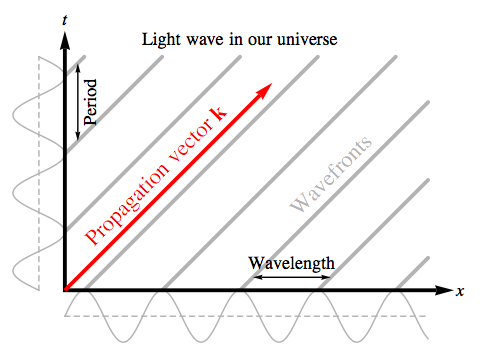The reciprocal of a wave’s frequency is the period of the wave, τ: the time it takes to complete one cycle. Because the sine function repeats itself exactly when its argument is increased or decreased by 2 π, our light wave A(x, y, z, t) will repeat itself when t is increased or decreased by τ = 1/ν:

A(x, y, z, t±τ) = A(x, y, z, t)

In units where the speed of light is 1, the light’s wavelength will be the same as its period: λ = 1/ν. Our wave A(x, y, z, t) will repeat itself when x is increased or decreased by one wavelength:

A(x±λ, y, z, t) = A(x, y, z, t)

Now, suppose we define a null vector, k, with components in our coordinate system of:

kx = 2 π ν
ky = 0
kz = 0
kt = 2 π ν

We will call this the propagation vector for our light wave. We can rewrite the wave’s amplitude as:

A(x, y, z, t) = A0 sin(kx x + ky y + kz zkt t)

The expression kx x + ky y + kz zkt t, where we multiply all the components of the propagation vector k by the corresponding components of x = (x, y, z, t), is reminiscent of the dot product we defined for use in Riemannian geometry; the only difference is the minus sign for the product of the t components. In fact it’s precisely the Lorentzian equivalent of k · x, and it’s a quantity that all observers in our Lorentzian universe would agree on, regardless of how they are moving.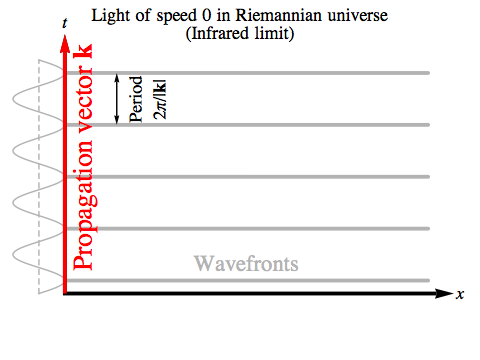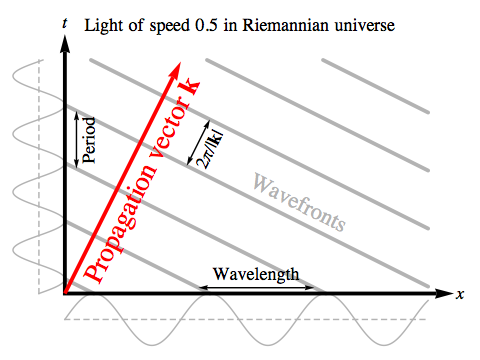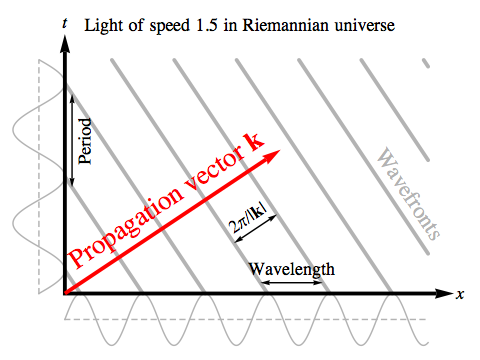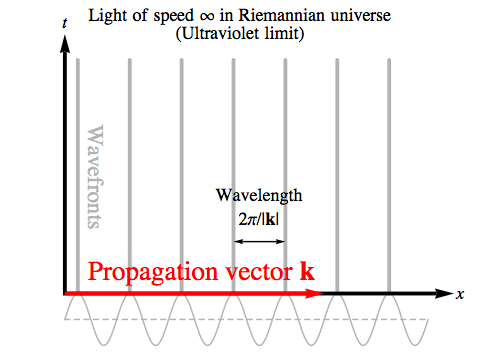This suggests that a similar kind of wave that moves through the vacuum in the Riemannian universe ought to take the form:

A(x, y, z, t) = A0 sin(k · x)

where this is the Riemannian dot product. In Riemannian geometry there are no null vectors; all directions in four-space are essentially the same. So on the face of it k could be any vector at all.

We can, however, always choose our coordinate system so that k lies in the xt plane. We will then have ky = kz = 0, and:

A(x, y, z, t) = A0 sin(k · x) = A0 sin(kx x + kt t)

The wavefronts, or “crests”, of this wave will occur wherever sin(k · x) = 1, i.e. wherever k · x = π/2 + 2nπ for some integer n. If we find one point where k · x takes such a value, in four-space there will be three directions perpendicular to k in which we can move without changing k · x at all, so the wavefronts will be a succession of regularly spaced three-dimensional regions, perpendicular to k. In our diagrams, we show a two-dimensional slice through four-space, and these regions show up in that slice as a sequence of parallel lines.

What are the wavelength λ and period τ of this wave? It’s not hard to see that:

A(x±(2 π / kx), y, z, t) = A(x, y, z, t)
A(x, y, z, t±(2 π / kt)) = A(x, y, z, t)

so the wavelength and period are:

λ = 2 π / kx
τ = 2 π / kt

We will call the reciprocal of the wavelength the spatial frequency, κ (the Greek letter kappa); this is precisely analogous to the time frequency ν, and tells us how many cycles of the wave fit into a unit distance. Then we can write the components of our propagation vector k as follows:

kx = 2 π / λ = 2 π κ
ky = 0
kz = 0
kt = 2 π / τ = 2 π ν

and we will have the following relationship between the spatial frequency, κ, the time frequency, ν, and the magnitude of the propagation vector, |k|:

|k|2 = (kx)2 + (kt)2 = (2 π κ)2 + (2 π ν)2

and so

κ2 + ν2 = |k|2 / (4 π2)

Now, observers moving with different velocities in the Riemannian universe will all agree on the value of |k|, the magnitude of the propagation vector, just as different observers in the Lorentzian universe will agree that the propagation vector associated with a light wave is a null vector. So we will assume that the particular physical phenomenon that’s the equivalent of light in the Riemannian universe will consist of waves that all share the same value for |k|.

The geometric effect of fixing |k| is to fix the separation between the wavefronts — not as measured in any particular observer’s chosen coordinate directions, but perpendicular to the wavefronts themselves, along the propagation vector. That separation in Riemannian space will always be equal to 2 π / |k|.

[We will later see that the value of |k| is related to the rest mass of the particle associated with these waves in the quantum mechanical version of the phenomenon, so suggesting that “all light has the same |k|” is really just insisting that we choose a particular particle to take the role the photon plays in our own universe. The photon has no rest mass, but in the Riemannian universe because there are no null vectors there are no particles without rest mass, so we have to choose some non-zero value.]

Once |k| is fixed, we can see that as the spatial frequency κ grows larger the time frequency ν must grow smaller, and vice versa, in order that the sum of their squares remains constant. If either of these frequencies is zero, the other will reach the maximum possible value, which we will call νmax. This is proportional to |k|.

νmax = |k| / (2 π)

The relationship between κ and ν becomes:

κ2 + ν2 = νmax2

As we have seen when we examined the Dual Pythagorean Theorem, this is just the relationship we’d expect between spatial frequencies measured along two perpendicular axes. In the Riemannian universe, the time frequency is no different from the frequency measured in any other dimension, so the mathematics is exactly the same. The frequency νmax is just the count of cycles of the wave per unit distance measured in the most direct way, perpendicular to the wavefronts in four-space.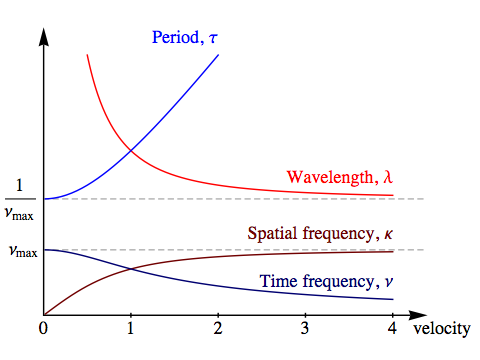Now, if we suppose that a pulse of light has a world line that follows the propagation vector, the velocity of the light, v, will equal the ratio between the x and t components of k:

v = kx / kt = κ / ν

The very slowest light, with v = 0, will have an infinitely long wavelength, λ = ∞. We’ll call this the “infrared limit”. The time frequency of this light will be νmax.

The very fastest light, with v = ∞, will have the smallest possible wavelength, λ = 1 / νmax. We’ll call this the “ultraviolet limit”. The time frequency of this light will be zero, giving it an infinitely long period.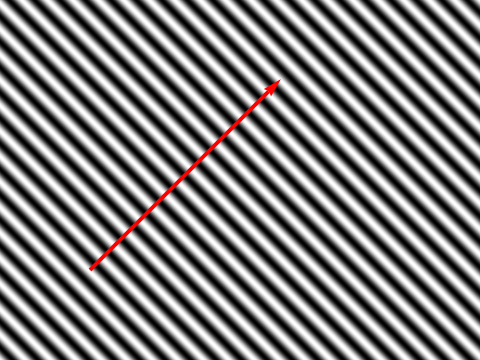But why do we suppose that a pulse of light has a world line that follows the propagation vector?

The waves we’ve been describing so far are plane waves: their wavefronts are infinite planes in space, which are slices of infinite hyperplanes in four-space. They are mathematically very simple, but obviously highly idealised — and a plane wave’s amplitude certainly isn’t concentrated into anything resembling a world line parallel to the propagation vector. The bright bands of maximum amplitude in the diagram on the right are perpendicular to the propagation vector, and over time these wavefronts are moving in the opposite direction to the propagation vector!

But these are infinite waves, occupying all of four-space. If we want a localised wave, we need to combine waves of different frequencies. The point where these waves reinforce each other will not move with the same velocity as any of the wavefronts of the contributing waves; its speed will be the group velocity of the system as a whole, not the phase velocity of the wavefronts.

We can find the group velocity for a combination of waves by looking at the relationship between x and t that will cause two plane waves of slightly different frequency to remain in phase. If the phases for two waves with spatial and time frequencies κ and ν for the first wave and κ+Δκ and ν+Δν for the second are to remain the same, we must have:

κx + νt = (κ + Δκ) x + (ν + Δν) t
x = – (Δν / Δκ) t
So vgroup = –dν/dκ

Applying this to what we know about the relationship between ν and κ:

ν = √(νmax2 – κ2)
vgroup = –dν/dκ = κ / √(νmax2 – κ2) = κ / ν

This agrees with the velocity kx / kt we obtained directly from the propagation vector.The image on the left was formed by adding together 61 plane waves in total (not all the arrows marking the propagation vectors are bright enough to see), and it provides a reasonable picture of the history of a pulse of light in the Riemannian universe. More realistically, a continuum of plane waves would be combined, rather than a finite number.

The result is roughly parallel to the average propagation vector, though it certainly isn’t a perfectly sharp world line — or even a “world tube” or “world strip” of constant width. But the fact that the pulse can be seen spreading out over time is exactly what we would expect: different frequencies of light travel at different velocities in the Riemannian universe, so any localised wave, built from many different frequencies, will gradually disperse this way.

### Scalar Waves

In the previous section we discussed the mathematical formulas for some very simple waves, but what we’d really like is an equation that all waves travelling through the vacuum in the Riemannian universe will satisfy, whatever their shape.

The equations satisfied by waves in our own universe are partial differential equations, which state some kind of relationship between the rates of change of the strength of the wave in various directions. If we have some quantity A that depends on several variables, such as the coordinates we’ve put on a region of four-space, x, y, z and t, then a partial derivative of A with respect to one of the variables is simply the rate of change of A when we change the variable in question and hold all the others fixed.

For example, consider a Riemannian plane wave with propagation vector k:

A = A0 sin(k · x) = A0 sin(kxx + kyy + kzz + ktt)

If we hold y, z and t fixed and vary x, A will oscillate in a sine wave. The rate of change of a sine function with respect to its argument is just the cosine of the same argument, but because the argument of the sine function here has x multiplied by a factor of kx, the rate of change of A is multiplied by that factor as well; this is just an example of a simple rule in calculus known as the chain rule.

When we take the partial derivative of some quantity A with respect to x, we will write this as ∂xA. This notation can be slightly intimidating if you haven’t used it before, but its meaning is really very simple: just imagine a line through space with all its coordinates fixed except x, and then think of how the quantity whose derivative you’re taking varies along that line, ignoring what it does elsewhere. Using this notation, we write:

xA = kx A0 cos(kxx + kyy + kzz + ktt)

What happens if we take the rate of change of this with respect to x? The rate of change of a cosine function with respect to its argument is minus the sine of the same argument, and again we get a factor of kx from the chain rule. We write a second partial derivative as ∂x2A, so:

x2A = –(kx)2 A0 sin(kxx + kyy + kzz + ktt) = –(kx)2 A

Now, the second partial derivatives with respect to the other three coordinates are:

y2A = –(ky)2 A
z2A = –(kz)2 A
t2A = –(kt)2 A

If we add up all four, we get:

x2A + ∂y2A + ∂z2A + ∂t2A
= –((kx)2 + (ky)2 + (kz)2 + (kt)2) A
= –|k|2 A

What we’ve done here is used the operation of taking the second rate of change of A in each coordinate direction as a means of multiplying the original function for the wave by a number proportional to the square of its frequency in that direction. Then by adding up all the second rates of change we get the sum of the squares, which equals |k|2, a factor that’s completely independent of the particular direction of the propagation vector.

Now |k| = 2 π νmax, but for the sake of brevity (to avoid having factors of 2 π everywhere) we will adopt the notation of “angular frequencies”, which are written with the symbol ω and are equal to 2 π times ordinary frequencies, ν. With this notation, |k| = 2 π νmax = ωm, and we can rewrite the last equation as:

 ∂x2A + ∂y2A + ∂z2A + ∂t2A + ωm2 A = 0 (RSW)

This equation makes no reference to any of the specifics of our original plane wave. All it refers to is a quantity A that varies over four-space, and a number ωm that is independent of the shape of the wave.

We will call this equation the Riemannian Scalar Wave (RSW) Equation. A “scalar” just means a number at each point in four-space that all observers can agree on, as distinct from, say, the components of a vector, which will depend on their choice of coordinate system. This equation is a four-dimensional version of an equation that occurs in the physics of our own universe, known as the Helmholtz equation.

The operation of taking a rate of change is linear: if s is a constant and A and B are functions of x:

x(s A) = sxA
x(A + B) = ∂xA + ∂xB

The same is true of second rates of change, and of course it’s also true of multiplication by a constant such as ωm2. So the RSW equation is a linear equation: if we take two solutions and add them, or multiply a solution by a constant, the result will be another solution. Since all Riemannian plane waves that share the same ωm satisfy the RSW equation, a wave formed by adding together any number of such plane waves will also satisfy it. For example, the wave we formed by adding together 61 plane waves at the end of the last section will satisfy the RSW equation.

There is one — potentially disastrous — problem with the RSW equation. Consider the wave:

A = sin[(5/4) ωm x] exp[(3/4) ωm t]

The rate of change of an exponential function is another exponential function, and the second rate of change gives you a positive multiple of the original function, unlike the negative multiple we get from sines and cosines. So we have:

x2A = –(25/16) ωm2A
y2A = 0
z2A = 0
t2A = (9/16) ωm2A

Adding up these terms, we see that the wave we’ve given here will satisfy the RSW equation. But what it describes is a wave that grows exponentially over time! The exponential factor is possible because the positive sign of its second rate of change is balanced by a spatial frequency greater than νmax in another coordinate direction: the angular frequency of (5/4) ωm in sin[(5/4) ωm x]. Simply saying that “νmax is the maximum possible frequency” doesn’t actually make it so, and the RSW equation alone is unable to enforce that restriction. But if waves like this are permitted, an initially minuscule disturbance of a high enough frequency will rapidly grow to overwhelm everything else.

There is a way to avoid this problem, but its discovery is an important part of the novel, so I’m not going to spoil the plot by disclosing it here.

### Vector Waves

Light in our own universe is an electromagnetic wave. Rather than being characterised by a single number that varies from place to place, the electromagnetic field involves vectors. So, we’d like to understand what kind of vector waves would make sense in the Riemannian universe.

Suppose we fix a four-vector, A0, with components A0x, A0y, A0z and A0t in our coordinate system. Then we can consider a vector plane wave:

A(x, y, z, t) = A0 sin(k · x)

This is very similar to the kind of scalar plane wave we considered earlier. In fact, each individual component of A in our coordinate system will satisfy the RSW equation, so long as |k| = ωm. Of course these components will be different in different coordinate systems ... but if they satisfy the RSW equation in one coordinate system they will do so in any other coordinate system. (Note that we’re talking here about different rectangular coordinate systems, not, say, spherical coordinates.)

That’s simple enough, but there’s a small complication we need to address. While the components of A, namely Ax, Ay, Az and At, change when we change coordinate systems, from the vector wave we’ve written above we can construct a scalar wave:

D(x, y, z, t) = k · A(x, y, z, t) = (k · A0) sin(k · x)

This wave is truly a scalar wave, in the sense that all observers will agree on its value, regardless of their choice of coordinate system. This might not seem important, but all our experience with physics in our own universe suggests that scalar and vector waves will be associated with distinct phenomena. In quantum mechanics, scalar waves are associated with particles with zero spin, while vector waves are associated with particles that possess a certain unit of spin – one example being photons. So we would expect the equivalent of light in the Riemannian universe to be a pure vector wave, with no scalar wave such as D coming along for the ride.

So, what we will require of a vector plane wave like A is that k · A0 = 0. Imposing this condition means that the vector describing the wave itself is orthogonal to the propagation vector, limiting the vector A0 to lie in a three-dimensional subspace orthogonal to k. Since that subspace is three-dimensional, Riemannian light will have three independent states of polarisation, as opposed to the two for light in our own universe. We will have a bit more to say about this when we examine Riemannian electromagnetism in detail.

We need to reformulate our “no scalar” condition into one we can impose on any vector function A(x, y, z, t). In the case of a plane wave A(x, y, z, t) = A0 sin(k · x) where we require k · A0 = 0, we see that:

x Ax + ∂y Ay + ∂z Az + ∂t At
= (A0x kx + A0y ky + A0z kz + A0t kt) cos(k · x)
= (k · A0) cos(k · x)
= 0

So we will declare that the following pair of equations constitute the Riemannian Vector Wave (RVW) Equations:

 ∂x2A + ∂y2A + ∂z2A + ∂t2A + ωm2 A = 0 (RVW) ∂x Ax + ∂y Ay + ∂z Az + ∂t At = 0 (Transverse)

where the first equation is telling us that all four individual components of A satisfy the RSW equation, while the transverse condition is a guarantee that the wave is a pure vector.

There is extra material on this topic for readers who don’t mind a slightly higher level of mathematics.Orthogonal / Geometry and Waves / created Wednesday, 6 April 2011